# QlikView Creating Analytics

Discussion Board for collaboration related to Creating Analytics for QlikView.Not applicable

## Sum doesn't "roll up" when collapsed

I have a table with 3 dimensions and two expressions. With the dimensions completely expanded, I get the values I'm looking for in "New business" and "Core business", but when they are collapsed, the sum of the Core and New business values don't roll up correctly. New Business gets rolled into Core: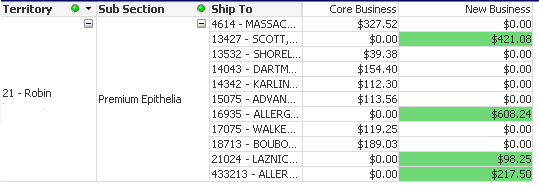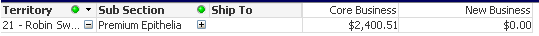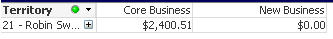Here are my two expressions:

Sum({\$*BM08 <[Fiscal Year]={'\$(FiscalCurrentYear)'},[Customer Sub Type]-={'ENT-Human'}>}[Sales Amount]) - Column(2)

if (Sum({\$*BM08 <[Fiscal Year]={'>=\$(=FiscalCurrentYear-2)<\$(FiscalCurrentYear)'},[Customer Sub Type]-={'ENT-Human'}>}[Sales Amount]) <= 0,

Sum({\$*BM08 <[Fiscal Year]={'\$(FiscalCurrentYear)'},[Customer Sub Type]-={'ENT-Human'}>}[Sales Amount]),0)

Can someone explain why this is happening and suggest how to fix it? Thanks in advance.

1 Solution

Accepted Solutions
Contributor III

## Re: Sum doesn't "roll up" when collapsed

I think it should work like you want (although probably a bit slow) if you nest the if inside the aggr

Something like this:

Sum(aggr(

If(Sum({\$*BM08 <[Fiscal Year]={'>=\$(=FiscalCurrentYear-2)<\$(FiscalCurrentYear)'},Month={'*'},[Customer Sub Type]-={'ENT-Human'}>}[Sales Amount])<=0,

Sum({\$*BM08 <[Fiscal Year]={'\$(FiscalCurrentYear)'},[Customer Sub Type]-={'ENT-Human'}>}[Sales Amount]),[Sales Catalog Sub Section],[Ship To])),0)

i might be slightly off with syntax, but give it a try.

8 Replies
Esteemed Contributor III

## Re: Sum doesn't "roll up" when collapsed

Why this is happening: I think that the "New Business" expression returns 0 in the collapsed mode because the condition returns false for the overall territory.
How to fix: If my assumption above is correct, you need not just a sum, but sum of sums grouped by dimensions, e.g.:
sum(aggr(expression, "Sub Section", "Ship To"))

The "Core Business" expressions probably will be correct after fixing the "New Business".

Regards,
MichaelNot applicable

## Re: Sum doesn't "roll up" when collapsed

Thank you so much for your reply Michael and PLEASE stick with me to get this figured out; I will be forever in your debt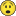Is this what you propose:

if (sum(aggr(Sum({\$*BM08 <[Fiscal Year]={'>=\$(=FiscalCurrentYear-2)<\$(FiscalCurrentYear)'},[Customer Sub Type]-={'ENT-Human'}>}[Sales Amount]) <= 0,[Sub Section],[Ship To])),

sum(aggr(Sum({\$*BM08 <[Fiscal Year]={'\$(FiscalCurrentYear)'},[Customer Sub Type]-={'ENT-Human'}>}[Sales Amount]),[Sub Section],[Ship To])),0)

I have tried it, but the results weren't correct and there was an immense amount of processing.Not applicable

## Re: Sum doesn't "roll up" when collapsed

I was able to get my expression working correctly. Unfortunately, when I collapse the dimensions, it still does not roll up. I've determined that this is caused by the IF statement. If I remove the IF and use only the expression, the values roll up (although incorrect). Is there a way to write the same expression without using the IF statement? Like with an e() in set analysis? Here is the expression:

If(Sum(aggr(Sum({\$*BM08 <[Fiscal Year]={'>=\$(=FiscalCurrentYear-2)<\$(FiscalCurrentYear)'},Month={'*'},[Customer Sub Type]-={'ENT-Human'}>}[Sales Amount]),[Sales Catalog Sub Section],[Ship To]))<=0,

Sum(aggr(Sum({\$*BM08 <[Fiscal Year]={'\$(FiscalCurrentYear)'},[Customer Sub Type]-={'ENT-Human'}>}[Sales Amount]),[Sales Catalog Sub Section],[Ship To])),0)

Contributor III

## Re: Sum doesn't "roll up" when collapsed

I think it should work like you want (although probably a bit slow) if you nest the if inside the aggr

Something like this:

Sum(aggr(

If(Sum({\$*BM08 <[Fiscal Year]={'>=\$(=FiscalCurrentYear-2)<\$(FiscalCurrentYear)'},Month={'*'},[Customer Sub Type]-={'ENT-Human'}>}[Sales Amount])<=0,

Sum({\$*BM08 <[Fiscal Year]={'\$(FiscalCurrentYear)'},[Customer Sub Type]-={'ENT-Human'}>}[Sales Amount]),[Sales Catalog Sub Section],[Ship To])),0)

i might be slightly off with syntax, but give it a try.Not applicable

## Re: Sum doesn't "roll up" when collapsed

THANK YOU! THANK YOU! THANK YOU! ANDERS!!! If I had a way to give you more points, I would. The syntax was just slighty off, but with a little bit of tweaking, it solved my problem. FINALLY!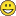Here is the final version:

Sum(aggr(

If(Sum({1*BM08 <[Fiscal Year]={'>=\$(=FiscalCurrentYear-2)<\$(FiscalCurrentYear)'},Month={'*'},[Customer Sub Type]-={'ENT-Human'}>}[Sales Amount])<=0,

Sum({\$*BM08 <[Fiscal Year]={'\$(FiscalCurrentYear)'},[Customer Sub Type]-={'ENT-Human'}>}[Sales Amount]),0),[Sales Catalog Sub Section],[Ship To]))

Thanks again!

Highlighted
Contributor III

## Re: Sum doesn't "roll up" when collapsed

You are most welcome. Glad i could help

Best wishes

Esteemed Contributor III

## Re: Sum doesn't "roll up" when collapsed

 venturebrowser wrote:... If I had a way to give you more points, I would. ...

You have this way now - you can give more points to Andres by marking his last reply as "helpful" 🙂

Regards,

MichaelNot applicable

Good idea. Done!